sinaplot: an enhanced chart for simple and truthful representation of single observations over multiple classes

Introduction

Recent developments in data science, in particular computational biology, often integrate data from several sources, over diverse experiments, or databases leaves a challenge of truthfully visualize data where the number of data points vary between classes. Plot types like bar charts, violin plot, strip charts or box-and-whiskers plots can provide visual information about mean/median, variance of the data, number of data points or density distribution of data; but only pairs of plots or dense overlays of these plot types will provide all the relevant information. To aid the presentation of datasets with differing sample size we have developed a new type of plot overcoming limitations of current standards visualization charts.

sinaplot is inspired by the strip chart and the violin plot. By letting the normalized density of points restrict the jitter along the x-axis the plot displays the same contour as a violin plot, but resemble a simple strip chart for small number of data points. In this way the plot conveys information of both the number of data points, the density distribution, outliers and spread in a very simple, comprehensible and condensed format.

Examples

x <- c(rnorm(200, 4, 1), rnorm(200, 5, 2), rnorm(400, 6, 1.5))
groups <- c(rep("Cond1", 200), rep("Cond2", 200), rep("Cond3", 400))

library(sinaplot)
sinaplot(x, groups)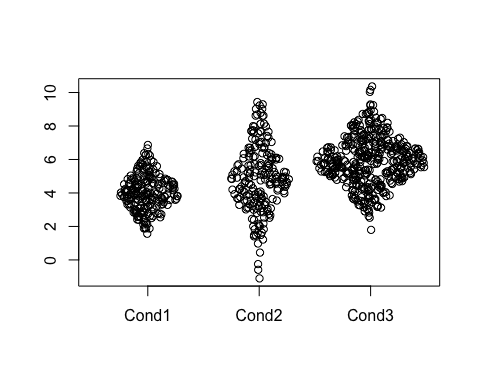#Use any "plot" argument
sinaplot(x, groups, col = 2:4, pch = 20, bty = "n")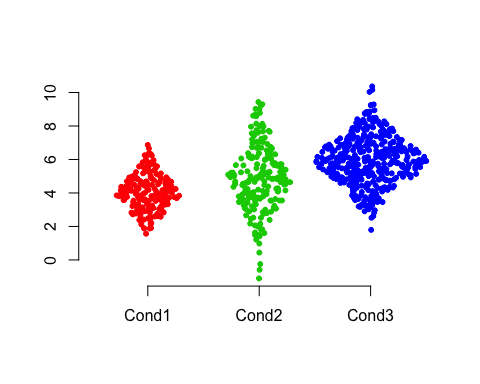Blood

We use a cohort of 2095 AML, ALL and healthy bone marrow samples to illustrate some of the strengths of sinaplot.

Class Gene
ALL t(12;21) 7.553129
ALL t(12;21) 7.252447
ALL t(12;21) 5.608201
ALL t(12;21) 5.971710
ALL t(12;21) 6.554109
ALL t(12;21) 5.655416
ALL t(12;21) 6.127554
ALL t(12;21) 6.043007
ALL t(12;21) 7.681021
ALL t(12;21) 5.959204

sinaplot(Gene ~ Class, data = blood, pch = 20)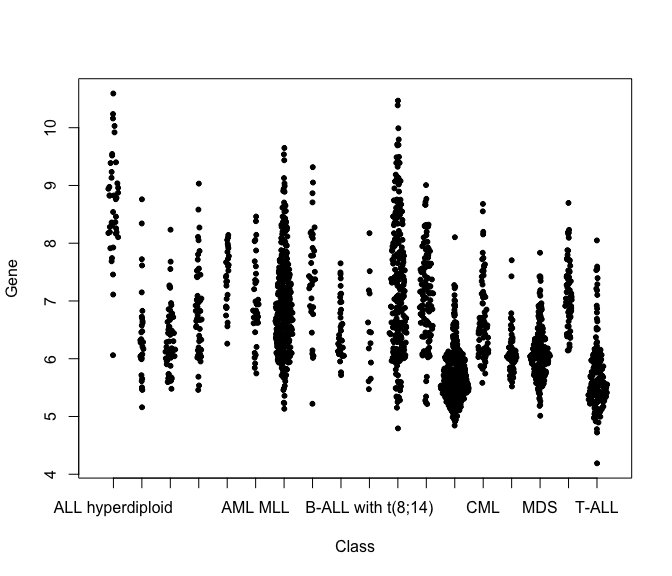By setting the argument scale = FALSE we turn off the group-wise scaling based on the class with the highest density.

sinaplot(Gene ~ Class, data = blood, pch = 20, scale = FALSE)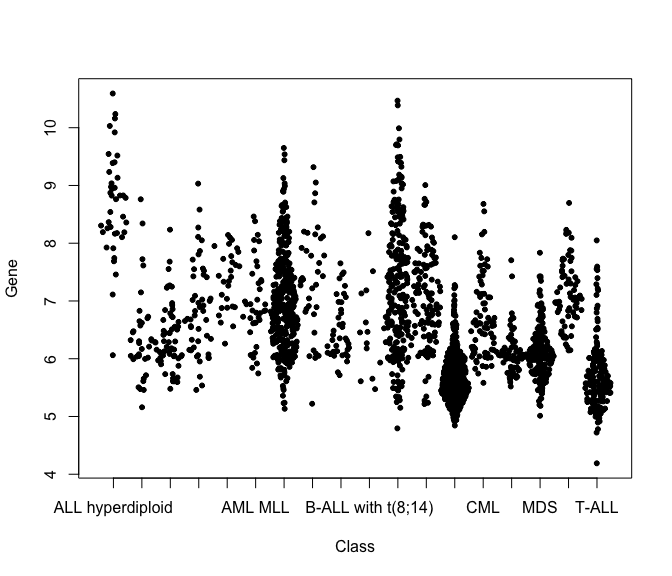Using the method = "counts" to compute the borders we get a less smooth spread of the samples due to the absence of the kernel density estimate.

sinaplot(Gene ~ Class, data = blood, pch = 20, scale = FALSE, method = "counts")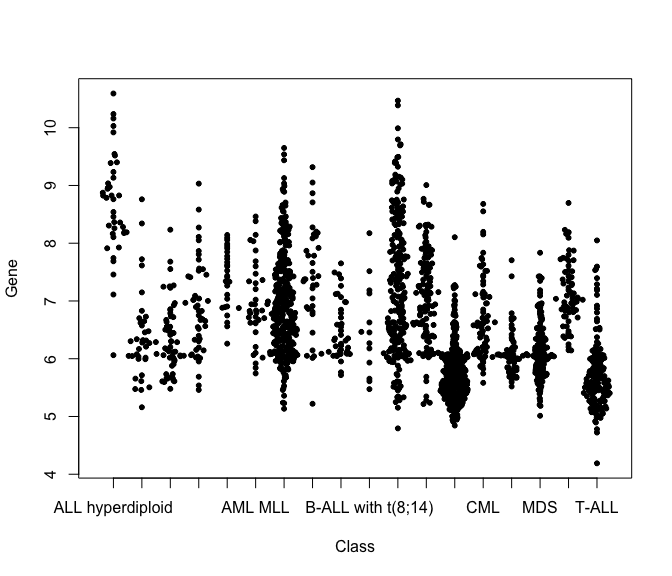Sinaplot aesthetics can be tweaked in the same manner as in graphics::plot.

par(mar = c(9,4,4,2) + 0.1)
n_groups <- length(levels(blood\$Class))

sinaplot(Gene ~ Class, data = blood, pch = 20, xaxt = "n", col = rainbow(n_groups),
ann = FALSE, bty = "n")
axis(1, at = 1:n_groups, labels = FALSE)
text(x = 1:n_groups,
y = par()\$usr - 0.1 * (par()\$usr - par()\$usr),
labels = levels(blood\$Class), srt = 45, xpd = TRUE, adj = 1,
cex = .8)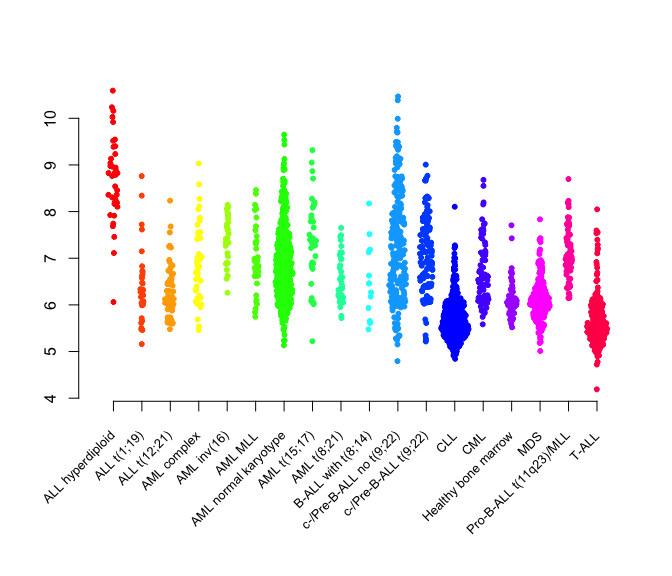Comparison

Using a subset of the blood dataset we compare sinaplot with 5 popular plotting strategies, and show how our package integrates features from these methods to achieve truthful and yet simple representation of multiclass single variable data.